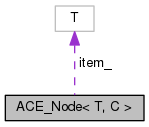ACE  6.3.2
ACE_Node< T, C > Class Template Reference

Implementation element in a Queue, Set, and Stack. More...

`#include <Node.h>`

Collaboration diagram for ACE_Node< T, C >:[legend]

## Public Member Functions

~ACE_Node (void)
This isn't necessary, but it keeps some compilers happy. More...

## Private Member Functions

ACE_Node (const T &i, ACE_Node< T, C > *n)

ACE_Node (ACE_Node< T, C > *n=0, int=0)

ACE_Node (const ACE_Node< T, C > &n)

void operator= (const ACE_Node< T, C > &)
Not possible. More...

## Private Attributes

ACE_Node< T, C > * next_
Pointer to next element in the list of ACE_Nodes. More...

item_
Current value of the item in this node. More...

## Friends

class ACE_Unbounded_Queue< T >

class ACE_Unbounded_Queue_Iterator< T >

class ACE_Unbounded_Queue_Const_Iterator< T >

class ACE_Unbounded_Set_Ex< T, C >

class ACE_Unbounded_Set_Ex_Iterator< T, C >

class ACE_Unbounded_Set_Ex_Const_Iterator< T, C >

class ACE_Unbounded_Stack< T >

class ACE_Unbounded_Stack_Iterator< T >

## Detailed Description

### template<class T, class C = void> class ACE_Node< T, C >

Implementation element in a Queue, Set, and Stack.

## Constructor & Destructor Documentation

template<class T , class C >
 ACE_Node< T, C >::~ACE_Node ( void )

This isn't necessary, but it keeps some compilers happy.

template<class T, class C>
 ACE_Node< T, C >::ACE_Node ( const T & i, ACE_Node< T, C > * n )
private
template<class T, class C>
 ACE_Node< T, C >::ACE_Node ( ACE_Node< T, C > * n = `0`, int = `0` )
private
template<class T, class C>
 ACE_Node< T, C >::ACE_Node ( const ACE_Node< T, C > & n )
private

## Member Function Documentation

template<class T, class C = void>
 void ACE_Node< T, C >::operator= ( const ACE_Node< T, C > & )
private

Not possible.

## Friends And Related Function Documentation

template<class T, class C = void>
 friend class ACE_Unbounded_Queue< T >
friend
template<class T, class C = void>
 friend class ACE_Unbounded_Queue_Const_Iterator< T >
friend
template<class T, class C = void>
 friend class ACE_Unbounded_Queue_Iterator< T >
friend
template<class T, class C = void>
 friend class ACE_Unbounded_Set_Ex< T, C >
friend
template<class T, class C = void>
 friend class ACE_Unbounded_Set_Ex_Const_Iterator< T, C >
friend
template<class T, class C = void>
 friend class ACE_Unbounded_Set_Ex_Iterator< T, C >
friend
template<class T, class C = void>
 friend class ACE_Unbounded_Stack< T >
friend
template<class T, class C = void>
 friend class ACE_Unbounded_Stack_Iterator< T >
friend

## Member Data Documentation

template<class T, class C = void>
 T ACE_Node< T, C >::item_
private

Current value of the item in this node.

template<class T, class C = void>
 ACE_Node* ACE_Node< T, C >::next_
private

Pointer to next element in the list of ACE_Nodes.

The documentation for this class was generated from the following files: